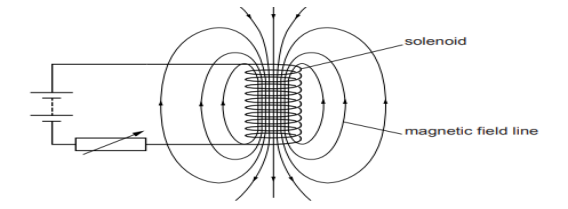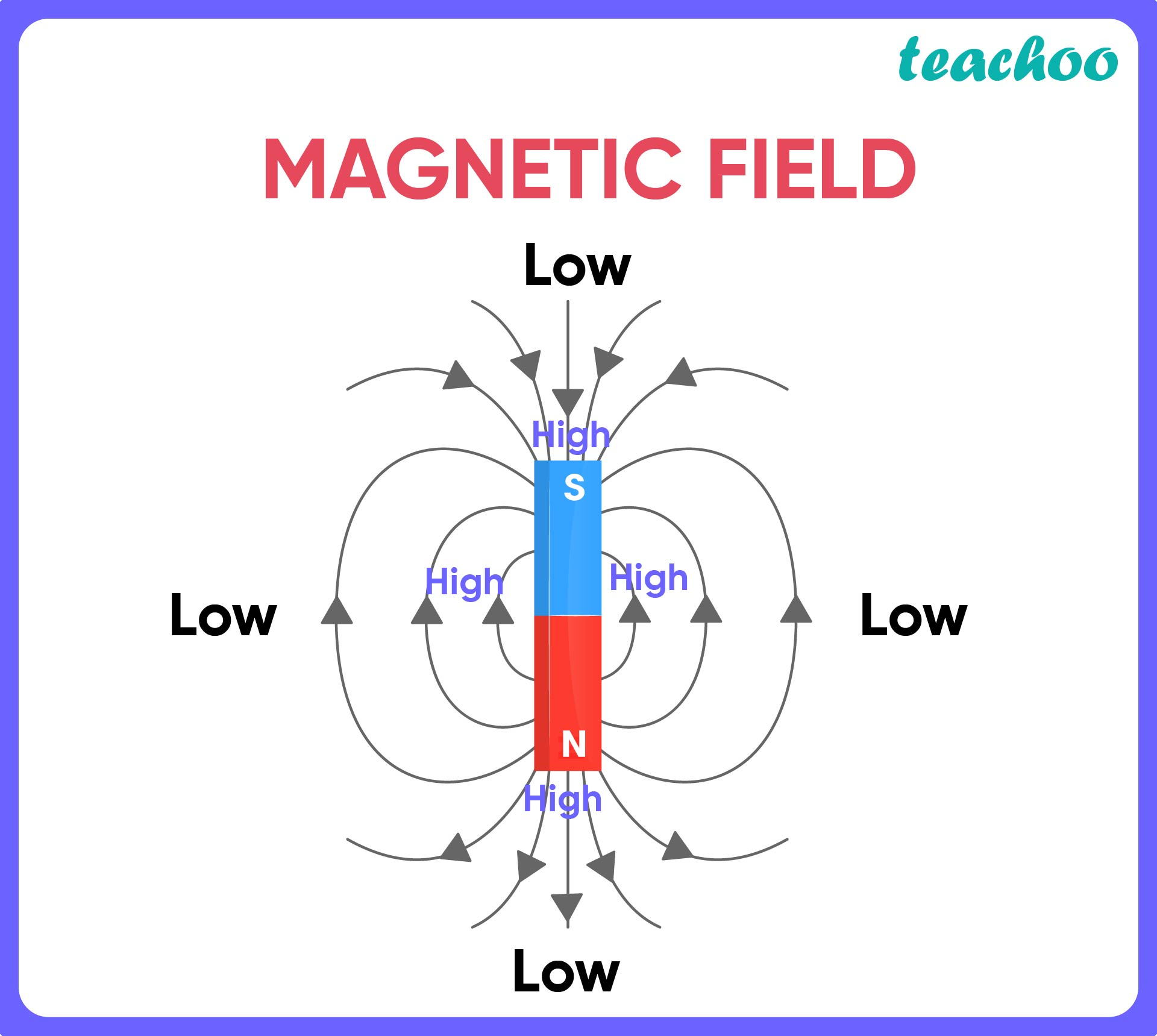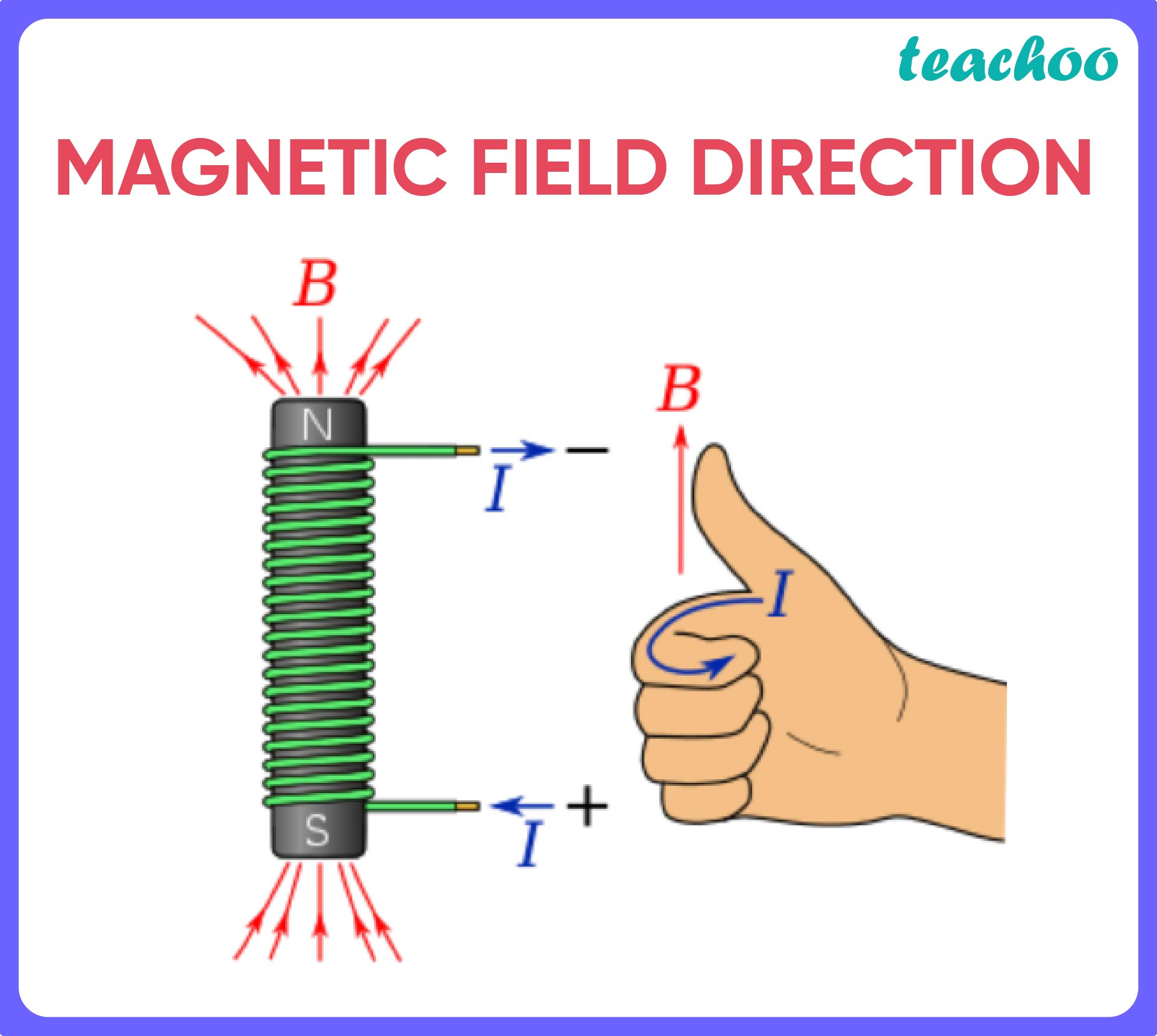CBSE Class 10 Sample Paper for 2022 Boards [Term 2] - Science Solution

Class 10
Solutions to CBSE Sample Paper - Science Class 10

## A circuit contains a battery, a variable resistor and a solenoid. The figure below shows the magnetic field pattern produced by the current in the solenoid.## b. What happens to the magnetic field when the current in the circuit is reversed?

A magnetic field pattern indicates the strength and direction of a magnetic field.

• The field is strong in places where the number of magnetic field lines per unit area is high .
• The field is lower in strength where the number of magnetic field lines per unit area is low .## b. What happens to the magnetic field when the current in the circuit is reversed?

The reversal of the current direction would change the direction of the magnetic field.

• Direction of a magnetic field in a solenoid is found using the following rule:Thus, if the direction of current is reversed, the direction of magnetic field is also reversed.

Get live Maths 1-on-1 Classs - Class 6 to 12